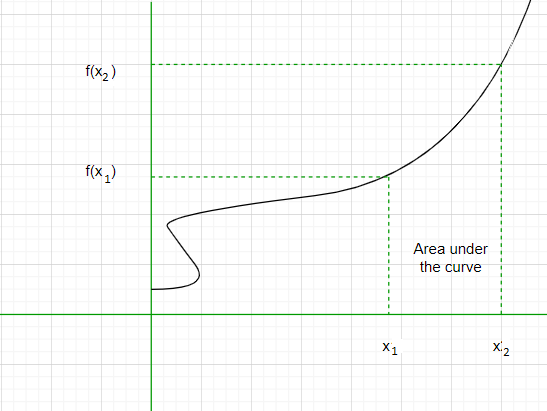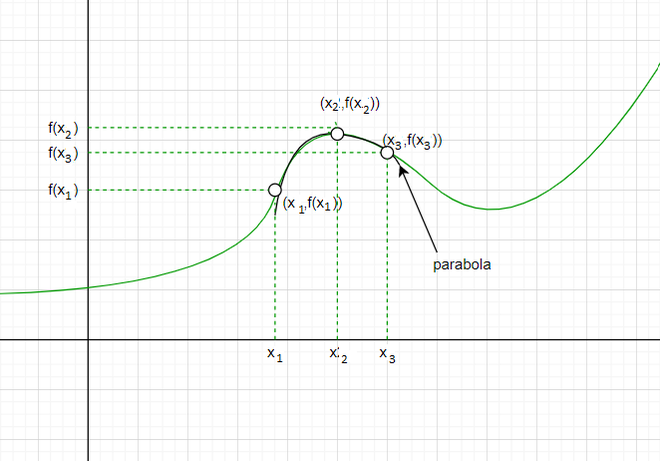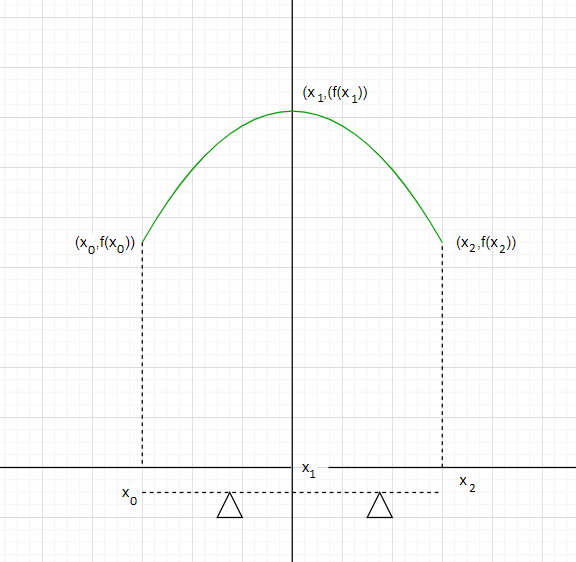Open in App
Not now

# Simpson’s Rule Formula

• Last Updated : 03 May, 2022

A way of finding the area of any figure is by integrating the function of that curve within the required limits which gives the area under that curve. However, there is a problem with this approach as in some cases the integral of the function can not be calculated or is difficult to find. It should also be noted that in many real-life engineering situations finding an approximate value of the area can be sufficient. This is where Simpson’s formula comes into the picture.The area under the curve

### Simpson’s Rule Formula

The Simpson’s rule formula is a mathematical formula given by British mathematician Thomas Simpson which is used for approximating the value of a definite integral. The rule states that :≈ Sn

Where Sn.

Here, a=x0 and b = xn, n = any even integer.

Derivation of Simpson’s rule formula

For a better understanding of how Simpson’s formula helps in approximating the area let’s derive the actual formula. Consider a function y = f(x) which is continuous in the closed interval [a, b]. We are going to approximate the value of integralby dividing the area under the curve into parabolas. For doing this divide the interval [a,b] into sub-intervals [x0, x1], [x1, x2], [x2, x3],…,[xn-2, xn-1], [xn-1, xn] each of width. Here x0 = a and xn = b. Now for finding the area let’s suppose that a parabola passes through every three consecutive points on the curve, i.e. there is a parabola that passes through points (x0, f(x0)), (x1, f(x1)), (x2, f(x2)).Parabola passing through three points taken out of the curve

Next we make this parabola symmetric along the y-axis. Lets assume the equation of this parabola to be px2 + qx + r.Parabola made symmetric along the y-axis

Now the area from x0 to x2 can be found by the definite integral(px2 + qx + r)dx..+0 +2r.

=⇢ (1)

Also, f(x0) =.

f(x1) =  r [since x1 = 0]

f(x2) =.

Now. ⇢  (2)

Compare (1) and (2),This means that, Area between x₀ and x₂ = f(x0​)+4f(x1​)+f(x2​)

Similarly we can find the area between the points x2 and x4. =.

We can now calculate the other areas in a similar way.Error bound in Simpson’s Rule

As mentioned earlier Simpson’s rule is helpful only for finding the approximate value of the integral. there is always an error bound in the calculation which is given byHere M>|f4(x)|

### Sample problems

Question 1: Find the integralfor n = 4

Solution:

Here f(x) = x4, a = 0, b = 4 and n = 4,.

By Simpson’s formula,.

= (1 + 4 + 32 + 324 + 256)/3 = 205.66

Note: This solution provided by Simpson’s formula is only an approximation the actual value of the integral will be 204.8. In later examples, we will see how this rule is helpful for finding the definite integral of functions whose integral we are unable to find.

Question 2: Find the integralfor n = 4

Solution:

Here f(x) = cos x a = 0, b = π, n = 4 and.

By Simpson’s formula.

= 0/3 = 0.

Question 3: Solve for the integralfor n = 2.

Solution:

Here f(x)=, a = 0, b = 2, n = 2 and= 2 – 0/2 = 1.

Using Simpson’s formula.

=.

= 2988.6762/3 = 995.8920

Note: The indefinite integral of the function, in this case, cannot be found. However, we could have used the limit of sums method for finding the integral but since it requires a lot more calculations, therefore, Simpson’s formula is preferred.

Question 4: Solve for the integralwhere n = 4.

Solution:

Here f(x) = ln x, a= e2, b = 2e2, n = 4 and \Delta= \frac{e^2}{4}.

Applying the Simpson’s formula.

=[2 + 4ln5 – 4ln4 + 8 + 2ln3 – 2ln2 + 4 + 4ln7 – 4ln4 + 8 + 2ln2 + 4].

= 15.3159106.

Question 5: Find the integralwhere n = 4.

Solution:

Here f(x) = sin2x, a = 0, b = π, n = 4 and= π/4.

Using Simpson’s formula.

= π/12[2 + 2 + 2]

= π/2

Question 6: Integrate the function x cos x between the limits 0 tousing Simpson’s rule and check the accuracy by calculating the actual integral. (Take n = 4).

Solution:

We need to find the integral.

Using Simpson’s formula for n = 4..

= 0.89

Now..– 1 = 0.57 .

So the difference between the Simpson value  and the actual  value of the integral = 0.89-0.57 = 0.32.

Question 7: Given a function f(x) = x cos (x) check the accuracy of Simpson’s rule formula for n = 2 and n = 4 within the limits 0 and π/2.

Solution:

Let’s check the value of the integral by using Simpson’s formula for n = 4.

= 0.8879

Now let’s apply the Simpson’s rule on the same integral but this time for n=2..

= 0.583

Now the value of the integral varies significantly as you change the value of n. The accuracy of the Simpson’s rule depends on the value of n. This shows that Simpson’s rule depends on the value of n it is mostly advised to take the value of n = 4.

My Personal Notes arrow_drop_up